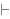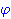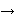Intuitionistic Logic Explorer < Previous   Next > Nearby theorems Mirrors  >  Home  >  ILE Home  >  Th. List  >  hbth Unicode version

Theorem hbth 1368
 Description: No variable is (effectively) free in a theorem. This and later "hypothesis-building" lemmas, with labels starting "hb...", allow us to construct proofs of formulas of the formfrom smaller formulas of this form. These are useful for constructing hypotheses that state "is (effectively) not free in." (Contributed by NM, 5-Aug-1993.)
Hypothesis
Ref Expression
hbth.1Assertion
Ref Expression
hbthProof of Theorem hbth
StepHypRef Expression
1 hbth.1 . . 321ax-gen 1354 . 232a1i 9 1Colors of variables: wff set class Syntax hints:wi 4wal 1257 This theorem was proved from axioms:  ax-1 5  ax-mp 7  ax-gen 1354 This theorem is referenced by:  nfth  1369  sbieh  1689  bj-sbimeh  10299
 Copyright terms: Public domain W3C validator